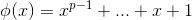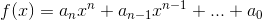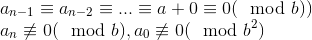# Abstract Algebra : Splitting Fields

## Example Questions

### Example Question #1 : Fields

What definition does the following correlate to?

Ifis a prime, then the following polynomial is irreducible over the field of rational numbers.Primitive Field Theorem

Principal Ideal Domain

Eisenstein's Irreducibility Criterion

Gauss's Lemma

Ideals Theorem

Eisenstein's Irreducibility Criterion

Explanation:

The Eisenstein's Irreducibility Criterion is the theorem for which the given statement is a corollary to.

The Eisenstein's Irreducibility Criterion is as follows.is a polynomial with coefficients that are integers. If there is a prime numberthat satisfy the following,Then over the field of rational numbersis said to be irreducible.

### All Abstract Algebra Resources